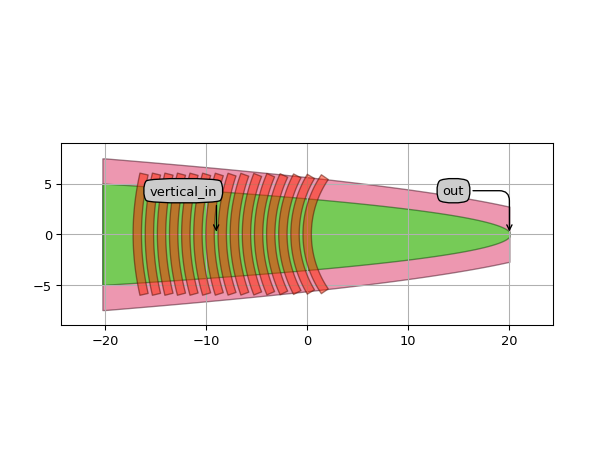# Grating coupler¶

SiFab contains an example grating coupler. Grating couplers come in many variations (straight, curved, 2D etc.). This demonstration grating coupler is built from scratch.

## GratingCoupler¶

This is a grating coupler with fully customisable layout. The GratingCoupler PCell is built hierarchically with two instances: one from the Socket and one from the Grating. The socket contains the waveguide elements and the related properties, and also knows about the in-plane port. The grating is also parameterized so users can specify e.g. grating period, line width, line length, number of periods, and curvature.

from si_fab import all as si_fab
from ipkiss3 import all as i3

fc = si_fab.GratingCoupler(
line_width=0.83,
period=1.2,
n_o_periods=15,
)
fc_lo = fc.Layout()

fc_lo.visualize(annotate=True)## FC_TE_1550¶

This is a specialization of the generic GratingCoupler PCell, which relates to the grating coupler compact model we defined in this PDK for 1550 nm wavelength.

from si_fab import all as si_fab
from ipkiss3 import all as i3
import numpy as np
import pylab as plt

fc = si_fab.FC_TE_1550()
fc_lv = fc.Layout()
fc_lv.visualize(annotate=True)

fc_cm = fc.CircuitModel()

wavelengths = np.linspace(1.54, 1.56, 1000)
S = fc_cm.get_smatrix(wavelengths=wavelengths)
plt.figure()
plt.subplot(121)
plt.plot(wavelengths, 10 * np.log10(np.abs(S["vertical_in", "out"]) ** 2), 'rx-')
plt.xlabel("Wavelengths ($\\mu$m)")
plt.ylabel("Transmission (dB)")
plt.title('power transmission')
plt.xlim([wavelengths, wavelengths[-1]])
plt.subplot(122)
plt.plot(wavelengths, np.angle(S["vertical_in", "out"]), 'bx-')
plt.xlabel("Wavelengths ($\\mu$m)")
plt.ylabel("Phase")
plt.title('phase transmission')
plt.xlim([wavelengths, wavelengths[-1]])
plt.show()